# Text Data Analysis of YouTube

In this article, I will let you know about the analysis of YouTube on basis of comments, likes, and dislikes. Simply we have to analyse the sentiment of the user based on the dataset.

Now, firstly let me tell you what is sentiment or what do you mean by sentiment. Sentiment is simply and emotion or a thought of a person or simply we can say that it is an opinion of one person that is expressed. A negative sentiment means some bad emotion or bad thought or some bad opinion. For example :- Let’s say that you have said a guy that you looks ugly means you have negative sentiment for that person. And similarly a positive sentiment is some good thought, good emotion or good opinion. Now let’s look at one example of positive sentiment, let’s say that you have met a person who looks very beautiful and you told him/her that you looks good, so this is a positive sentiment.

I hope that you understand till this point what is positive sentiment and what is negative sentiment. Now let’s start working with dataset. I am using Jupyter Notebook for this project, you can also use it otherwise you can use Google Colab for the same project. So firstly, you have to import and load all the necessary libraries for that.

# Importing the Dataset

Now after loading all the libraries you need to load the dataset. As I have taken dataset from Kaggle, so you can also take it from here :- Click Here. Now we can load the dataset using the following lines of code :-

After loading let’s see what does it contains and see what’s inside the dataset :-

# Looking into the dataset

Now we have to perform the sentiment analysis on the basis of comment_text feature given in the dataset. And to do that sentiment analysis we have to install a package called “textblob”. For loading it we can either use the anaconda prompt (that looks like command prompt) or we can use the jupyter notebook itself. As I am loading it using Jupyter Notebook, let me show you how to load the textblob in the jupyter environment.

# Installing the TextBlob library

Now after loading this we have to import it like this :-

Now after this we will try to check whether the statement is a positive statement and to do this we will check the polarity of any sentence. Polarity simply defines the orientation of the expressed statement, i.e.; if the sentence determines positive sentiment then the value of the polarity will be above 0 and less than or equal to 1 and if the sentence determines negative sentiment then the value of the polarity will be below 0 and greater than or equal to -1. And if the statement determines neutral sentiment then the value of polarity will be between -1 and 1. Let me tell you one more thing that the value of polarity lies in the range of [-1,1] where 1 means positive statement and -1 means a negative statement.

# Main Tasks in this Project

Now, let me tell you that our task here is :-

1. Performing sentiment analysis on YouTube comments.
2. Performing exploratory data analysis on positive sentences
3. Performing exploratory data analysis on negative sentences.

# Cleaning the Dataset

## Checking for missing values

Till this point, we have loaded the dataset and all the libraries. Now, here comes the part to find whether there is any missing values in the dataset or not. And we can do the same using this code :-

## Removing the missing values from the dataset

This shows that we have 28 missing values in the column comment_text. So, we will simply drop those 28 values using the following code :-

Now as the missing values are dropped let’s again check whether it reflects in the original dataset.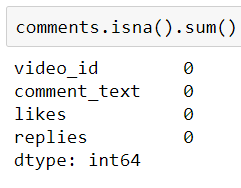Now there is no missing values.

## Storing all sentiment of sentence into a variable

Here we have no missing values. So we will simply start checking the polarity of each statement in the column “comment_text” and store that polarity values in a list using the following code :-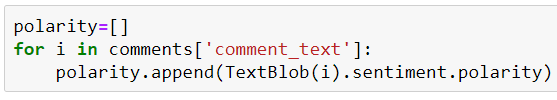We have stored the polarity values in the list named polarity.

Now we can add a new column in the dataset and name it as polarity and add all the values stored in the list named polarity using the following code :-

# Updating the comments dataset

This will create a new column named as “polarity” in the dataset comments. Now we can cross check our dataset using .head() command and see whether all the polarity values have been uploaded or not.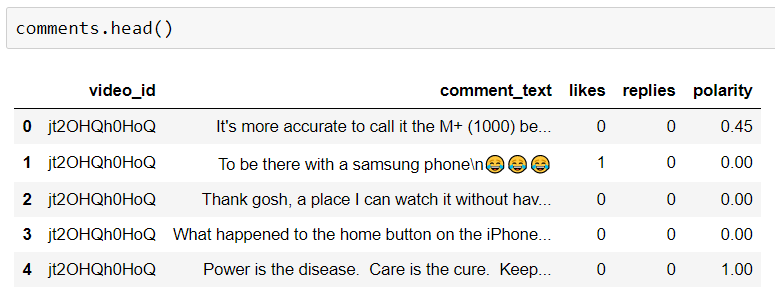Here we can see that polarity values have been updated in the dataset and the column name is polarity.

Now till here we have analysed the sentiment of the sentence. So our first task of performing sentiment analysis is done till here. Now, we have to look to our next task i.e.; to perform exploratory data analysis on negative and positive sentences. Let’s first look at exploratory data analysis on positive sentences.

# Looking into the positive sentences

First we will make a dataframe named as comments_positive and store all the positive sentences data in that using the following lines of code :-

Now we’ll check the rows and columns in the comments_positive dataset by using this code :-

And get to know that there are 20400 rows and 5 columns and now let’s see the comments_positive dataset using the .head() command :-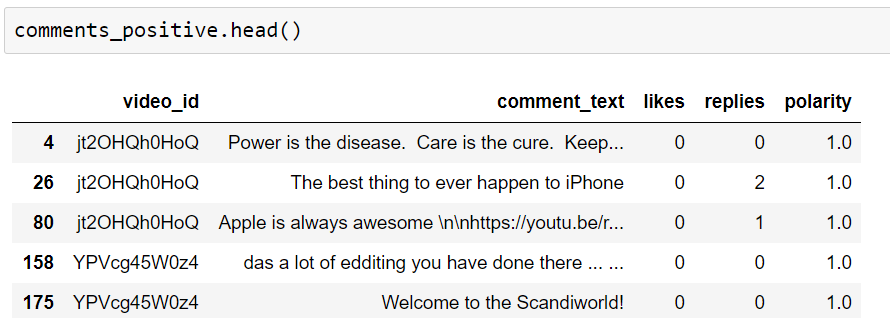Here you can see that all sentences having the polarity = 1

Now, we’ll visualize the same using the word cloud. Word cloud simply is a data visualization technique used for representing text data and from which we can get to know that if the size of word is bigger that means it has high frequency and more used. The size of the word indicates its frequency or importance. We can simply understand this as, it is a tool that tells how important our word in some huge chunks of data. More bigger the word, more important the word is.

# Installing the wordcloud

We can install the wordcloud using the following code :-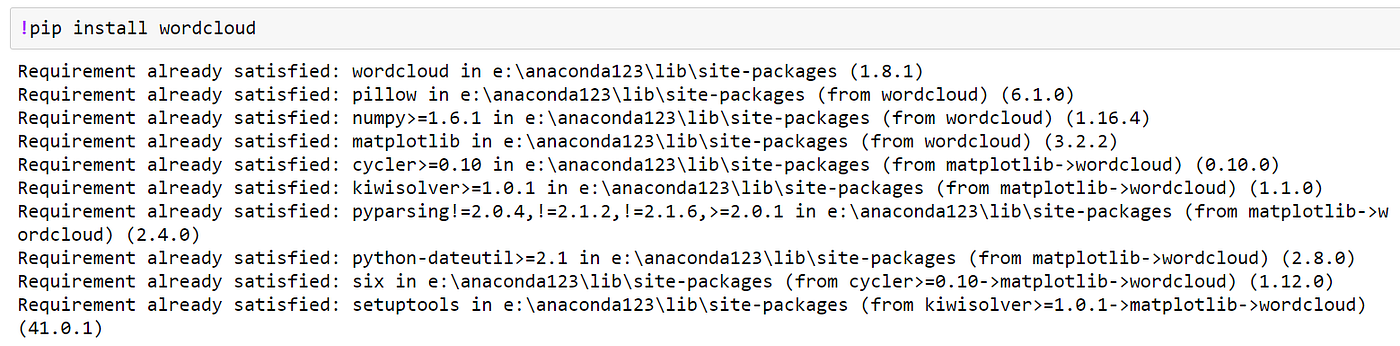As it is already installed in my PC, it is showing requirement already satisfied.

After installing, we have to simply import the wordcloud and stopwords, where stopwords are the words that are simply used in any language like the words :- “the”, “is”, “and” etc. these are the examples of stopwords which doesn’t make any sentiment in our sentence so we have to remove this from our sentence while making a WordCloud. Stopwords are used to eliminate unimportant words, due to which we can focus mainly on important and useful words.

Here is how we can import the wordcloud and stopwords :-

After importing necessary libraries we will assign stopwords the value of STOPWORDS, using the following code :-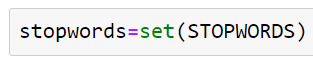This will take the unique words that are unimportant to us.

This will take the unique words that are unimportant to us that’s why we’ve taken here set to take only the unique words and assigned it to variable named stopwords.

After this, I have taken all the sentences that are in the comment_text into a variable and named it as total_comments using the following code :-

This code will store all the sentences that are individual stored in the column comment_text.

After this, we will form the WordCloud using the following lines of code :-

Here we have set the width as 1000 and height as 500 and we will assign the stopwords parameters as the words that are unimportant to us and we have already stored that in the variable named stopwords.

# Visualizing using WordCloud for positive sentences

Now, we will simply show the wordcloud using the following lines of code :-

Now, similarly we have to do the same thing for analyzing the negative sentences.

# Looking into negative sentences

Now, we will take all the sentences having polarity as -1 and store them in a variable called comments_negative using the following lines of code :-

Now, we will merge all the comments into one and name it as total_comments, using the following line of code :-

After this, we will form the wordcloud using the following lines of code :-

# Visualizing using WordCloud for negative sentences

After this, we will simply show the wordcloud using the following lines of code :-

# Closing Thoughts

That’s it for this project, till then enjoy coding and making projects using Python. And please let me know if you are stuck in between, I will definitely look into your problem.